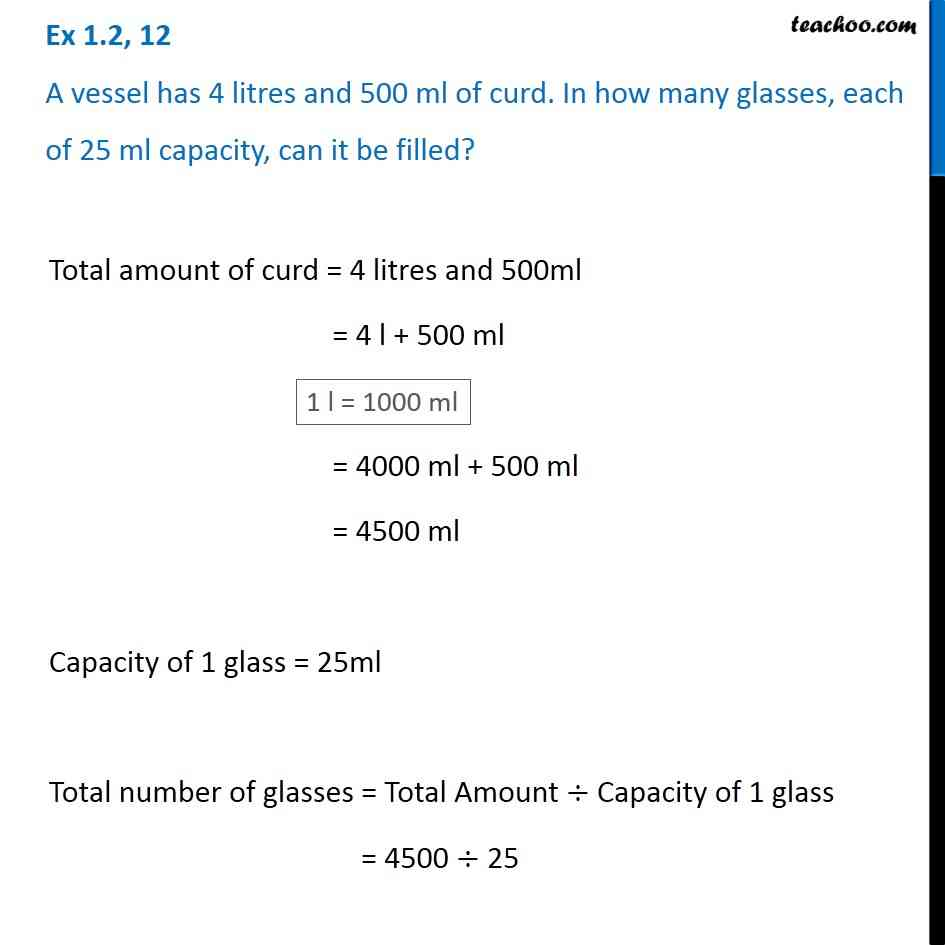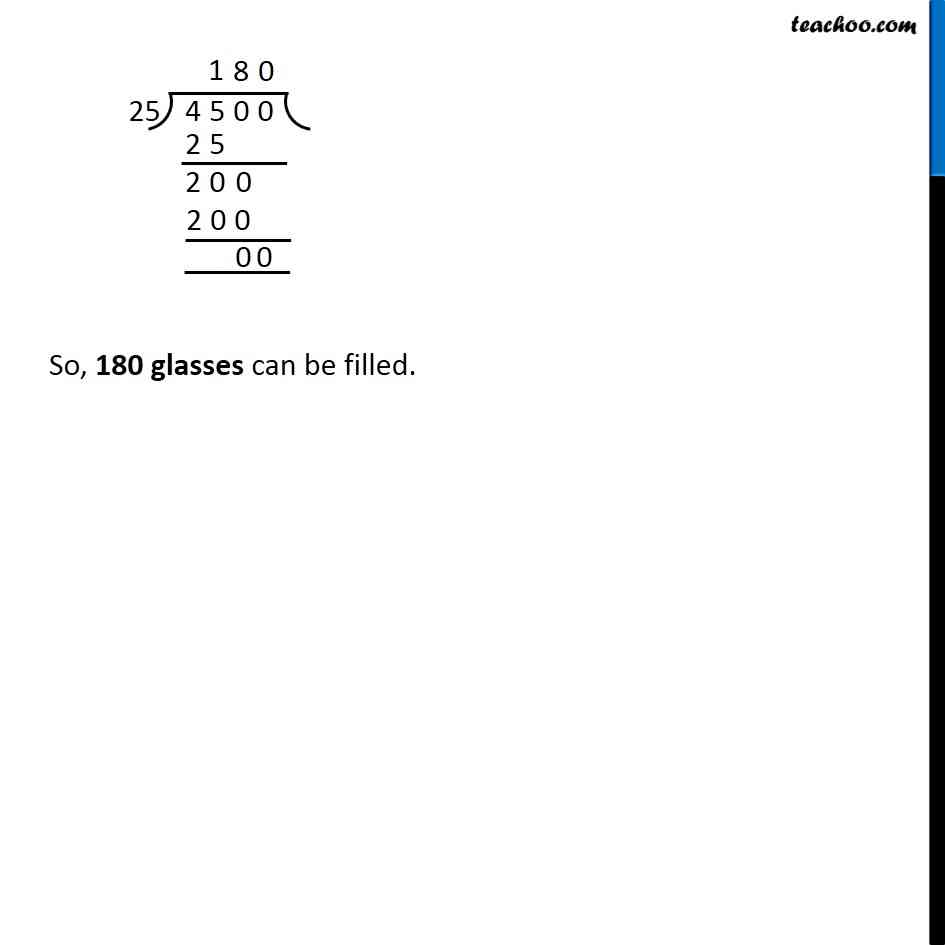1. Chapter 1 Class 6 Knowing our Numbers
2. Serial order wise
3. Ex 1.2

Transcript

Ex 1.2, 12 A vessel has 4 litres and 500 ml of curd. In how many glasses, each of 25 ml capacity, can it be filled?Total amount of curd = 4 litres and 500ml = 4 l + 500 ml = 4000 ml + 500 ml = 4500 ml Capacity of 1 glass = 25ml Total number of glasses = Total Amount ÷ Capacity of 1 glass = 4500 ÷ 25 So, 180 glasses can be filled.